# Digital Signal Processing Filter Design Examples

IIR Design -   Convert the band-pass analog filter with transfer function: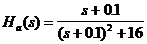which has a resonance (peak frequency response) at  W = 4 to a digital filter using the bi-linear transformation.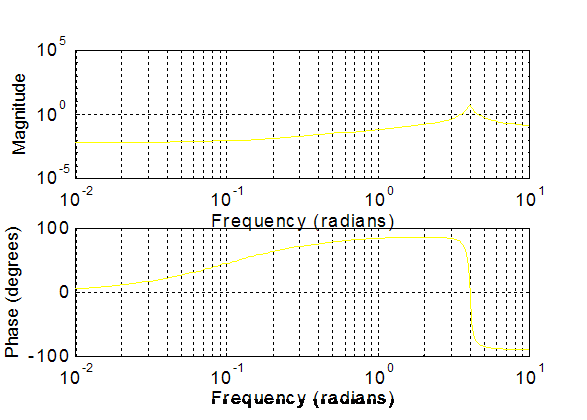The digital filter is to have a resonance at w = p/2

A.        What is the desired mapping?

Td = ½ to map  W = 4  to  w = p/2  using: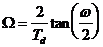or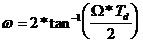The mapping is therefore: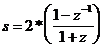B.         What is the resulting H(z)?

The resulting H(z) is: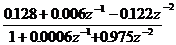C.        Where are the poles and zeros of this filter?

Factoring the numerator gives the zeros and factoring the denominator yields the poles.  The poles are approximately at 0.987e±jp/2 and the zeros are at -1 and 0.95

D. The Frequency Response of the digital filter (at a 1 kHz sample rate) is: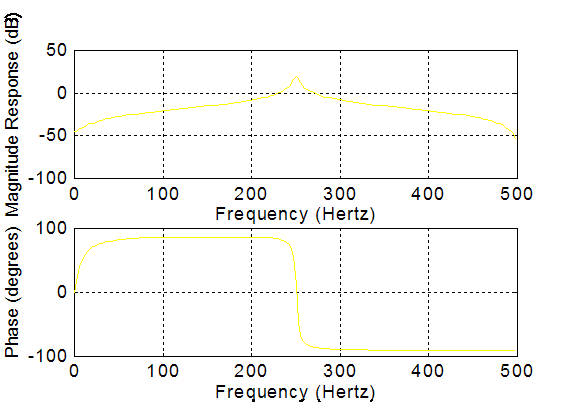FIR Design -  Starting with the ideal low-pass filter function with cutoff Wc = p/4 , develop an FIR low pass filter using a Hamming window that has the following characteristics:

wc = p/4           (cut off frequency)

M = 31                        (Filter length)

A.        What is the FIR filter pulse response?  Sketch it below.

The pulse response is the product of sin(x)/x (the Inverse Fourier Transform of the ideal filter shifted to center at n=16) and the Hamming raised cosine window function.B.         What is the approximate transition width of the resulting filter?

The transition width for the rectangular window would be 1/T in normalized frequency, with the Hamming Window the Transition width is doubled to 2/T in normalized frequency.

C.    Sketch the FIR filter frequency response below.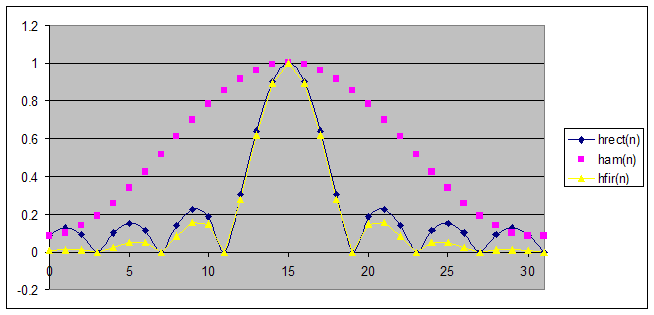This is a low pass filter.  The positive frequencies start at index 1 and go to index 16.  The highest negative frequency is at index 17.  DC (f=0) is at index 0 and 32.